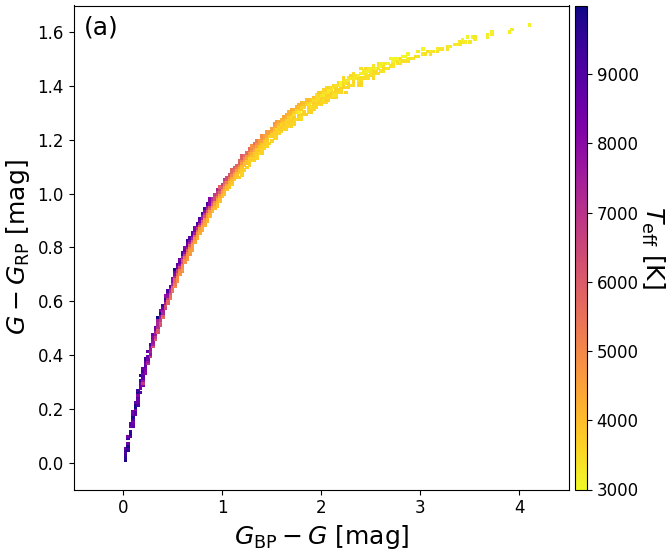# 8.1.1 What’s been done in Gaia DR2

Author(s): Coryn Bailer-Jones

We use the three-band photometry and parallaxes, together with various training data sets, to estimate the effective temperature $T_{\rm eff}$, line-of-sight extinction $A_{\rm G}$ and reddening $E(G_{\rm BP}-G_{\rm RP})$, luminosity ${\cal L}$, and radius ${\cal R}$, of up to 161 million stars brighter than $G$ =17; a total of five parameters. (Subsequent filtering removes parameters for some sources.) Although photometry for fainter sources is available in Gaia DR2, we chose to limit our analysis to brighter sources on the grounds that, at this stage in the mission and processing, only these give sufficient photometric and parallax precision to obtain reliable astrophysical parameters. The choice of 17 is somewhat arbitrary, however. The three broad photometric bands (see Section 5), provide relatively little information for deriving the intrinsic properties of the observed Gaia targets. Moreover, the $G$-band flux is nearly degenerate with the sum of the other two (see Figure 5.9). We therefore assume all sources to be single stars. A source-by-source classification to identify quasars, galaxies, and – to some extent – unresolved binary stars will be performed using the dispersed BP/RP spectra for Gaia DR3 (Bailer-Jones, C. A. L. et al. 2013). There will, of course, be relatively few extragalactic point sources in our sample of $G\leq 17$.

Stellar parameters are estimated source-by-source. We do not make use of any global Galactic information such as an extinction map or kinematics. We also do not use any non-Gaia data on the individual sources. We only use the three Gaia photometric bands (for $T_{\rm eff}$) and additionally the parallax (for the other four parameters).Figure 8.1: Colour–colour diagrams for stars from the PARSEC 1.2S models with an extinction law from Cardelli et al. (1989). While Teff is the dominant factor (panel a), it is strongly degenerate with extinction (panel b). (Andrae et al. 2018).

As can be seen in Figure 8.1, $T_{\rm eff}$ is heavily degenerate with $A_{\rm G}$, making it impossible to estimate both with any useful precision from these data alone. We therefore estimate $T_{\rm eff}$ from the colours on the assumption that extinction is low. This is done with a machine learning algorithm trained empirically: the training data are observed Gaia photometry of stars which have had their $T_{\rm eff}$ estimated from other sources. This training data set only includes stars which are believed to have low extinctions. This use of empirical training sets avoids biases which can occur when training on synthetic data (which occur due to inevitable mismatch between synthetic templates and real spectra).

We then separately estimate the interstellar absorption using the three fluxes together with the parallax (again using a machine learning algorithm). The signal here is the dimming of the sources due to absorption, as opposed to reddening. For this we train the algorithm on synthetic stellar photometry, because there are too few stars with reliably estimated extinctions which could be used as an empirical training set. The relative extinction parameter, $R_{\rm 0}$, is fixed to 3.1. Note that the absorption we estimate is the extinction in the G-band, $A_{\rm G}$, which is not the same as the (monochromatic) extinction parameter, $A_{\rm 0}$. The latter depends only on the amount of absorption in the interstellar medium at the single wavelength 547.7nm, whereas the former depends also on the spectral energy distribution (SED) of the star (see section 2.2 of Bailer-Jones 2011). Thus even with fixed $R_{\rm 0}$ there is not a one-to-one relationship between $A_{\rm 0}$ and $A_{\rm G}$, although in practice the scatter about a constant relation is small. For this reason we use a separate machine learning model (with the same inputs and trained with the same synthetic spectra) to estimate the reddening $E(G_{\rm BP}-G_{\rm RP})$, even though the available signal is still primarily the dimming due to absorption. Given the strong degeneracy between $T_{\rm eff}$ and $A_{\rm G}$ and $E(G_{\rm BP}-G_{\rm RP})$, respectively, our results contained many outliers and the decision was made to filter these out of Gaia DR2. Therefore, estimates of $A_{\rm G}$ and $E(G_{\rm BP}-G_{\rm RP})$are available for only 87.7 million sources.

We estimate the absolute G-band magnitude via (with r expressed in pc)

 $M_{G}\,=\,G+5-5\log_{10}r-A_{\rm G}$ (8.1)

This is converted to a stellar luminosity using the zeropoint of the $G$-magnitude system and a bolometric correction (see Section 8.3.3). The distance $r$ to the target is taken simply to be the inverse of the parallax in arcsec. Although this generally gives a biased estimate of the distance (see for example Bailer-Jones 2015), the impact of this is mitigated here by the fact that we only derive luminosities when the fractional parallax uncertainty $\sigma_{\varpi}/\varpi$ is less than 0.2. Thus of the 161 million stars with $T_{\rm eff}$ estimates, only 77 million have luminosity estimates included in Gaia DR2.

Having inferred the luminosity and temperature, the stellar radius is then given by the Stefan–Boltzmann law

 ${\cal L}\,=\,4\pi\sigma{\cal R}^{2}T_{\rm eff}^{4}$ (8.2)

Because our individual extinction estimates are rather poor for most stars (discussed later), we chose not to use them in the derivation of luminosities, i.e. we set $A_{\rm G}$ to zero in Equation 8.1. Consequently, while our temperature, luminosity, and radius estimates are self-consistent (within the limits of the adopted assumptions), they are formally inconsistent with our extinction and reddening estimates.